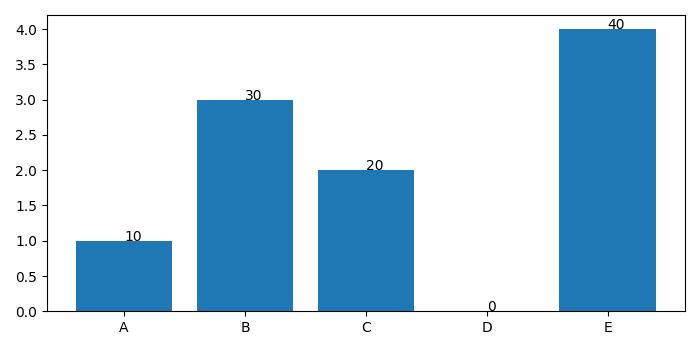# How can I display text over columns in a bar chart in Matplotlib?

MatplotlibPythonData Visualization

To display text over columns in a bar chart, we can use text() method so that we could place text at a specific location (x and y) of the bars column.

## Steps

• Create lists for x, y and percentage.

• Make a bar plot using bar() method.

• Iterate zipped x, y and percentage to place text for the bars column.

• To display the figure, use show() method.

## Example

import matplotlib.pyplot as plt
plt.rcParams["figure.figsize"] = [7.00, 3.50]
plt.rcParams["figure.autolayout"] = True
x = ['A', 'B', 'C', 'D', 'E']
y = [1, 3, 2, 0, 4]
percentage = [10, 30, 20, 0, 40]
ax = plt.bar(x, y)
for x, y, p in zip(x, y, percentage):
plt.text(x, y, p)
plt.show()

## Output